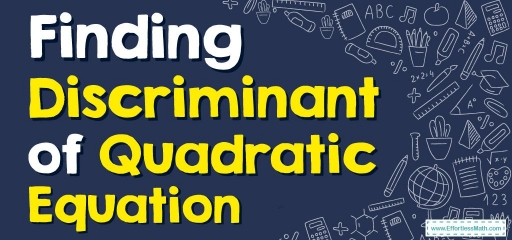# How to Find Discriminant of Quadratic Equation?The discriminant in mathematics is defined for polynomials and is a function of polynomial coefficients. Expresses the nature of the roots, or in other words, it discriminates against the roots.

## A step-by-step guide tofinding discriminant of quadratic equation

Discriminant of a polynomial in math is a function of the coefficients of the polynomial. This is useful in determining the type of solutions to a polynomial equation without actually finding them. That is, it distinguishes the solutions of the equation (equal and unequal; real and unreal) and hence it is called “discriminant”. The value of the discriminant can be any real number (i.e., either positive, negative, or $$0$$).

### How to find discriminant?

To find the discriminant of a quadratic equation, we just need to compare the given equation with its standard form and determine the coefficients.

#### Discriminant of a quadratic equation

The discriminant of a quadratic equation $$ax^2+bx+c = 0$$ is in terms of its coefficients $$a, b$$, and $$c$$. i.e.,

$$\color{blue}{Δ\:Or\:D = b^2− 4ac}$$

Do you remember using $$b^2 – 4ac$$ before? Yes, it is a part of the quadratic formula: $$x=\frac{-b\pm \sqrt{b^2-4ac}}{2a}$$. Here, the expression that is inside the square root of the quadratic formula is called the discriminant of the quadratic equation.  The quadratic formula in terms of the discriminant is $$x=\frac{-b\pm \sqrt{D}}{2a}$$.

### Discriminant, and nature of the roots

The roots of a quadratic equation $$ax^2+bx+c = 0$$ are the values of $$x$$ that satisfy the equation. They can be found using the quadratic formula: $$x=\frac{-b\pm \sqrt{D}}{2a}$$. Although we can not find the roots just by using discriminant, we can determine the nature of the roots as follows.

#### If the discriminant is positive:

If $$D>0$$, the quadratic equation has two different real roots. This is because, when $$D>0$$, the roots are given by $$x=\frac{-b\pm \sqrt{Positive\:number}}{2a}$$, and the square root of a positive number always results in a real number. So when the discriminant of a quadratic equation is greater than $$0$$, it has two roots that are distinct and real numbers.

#### If the discriminant is negative:

If $$D< 0$$, the quadratic equation has two different complex roots. This is because when $$D< 0$$, the roots are given by $$x=\frac{-b\pm \sqrt{Negative\:number}}{2a}$$, and the square root of a negative number leads to an imaginary number always. Therefore when the discriminant of a quadratic equation is less than $$0$$, it has two roots which are distinct and complex numbers (non-real).

#### If the discriminant is equal to zero:

If $$D = 0$$, the quadratic equation has two equal real roots. In other words, when $$D=0$$, the quadratic equation has only one real root. This is because, when $$D= 0$$, the roots are given by $$x=\frac{-b\pm \sqrt{0}}{2a}$$, and the square root of a $$0$$ is $$0$$. Then the equation turns into $$x= -\frac{b}{2a}$$ which is only one number. So when the discriminant of a quadratic equation is zero, it has only one real root.

### Finding Discriminant of Quadratic Equation– Example 1:

Find the discriminant of the following equation $$3x^2+10x−8$$.

Solution:

The given quadratic equation is $$3x^2+10x−8$$. Comparing this with $$ax^2+bx+c= 0$$, we get $$a =3, b = 10$$, and $$c =-8$$.

$$D = b^2- 4ac$$
$$=(10)^2- 4(3)(-8)$$
$$=100 + 96$$
$$= 196$$

## Exercises for Finding Discriminant of Quadratic Equation

### Find the discriminant of the following equation.

1. $$\color{blue}{3x^2+4x-8=0}$$
2. $$\color{blue}{5x^2+3x+1=0}$$
3. $$\color{blue}{6x^2-x-15=0}$$
4. $$\color{blue}{4x^2+4x+1=0}$$
1. $$\color{blue}{112}$$
2. $$\color{blue}{-11}$$
3. $$\color{blue}{361}$$
4. $$\color{blue}{0}$$

### What people say about "How to Find Discriminant of Quadratic Equation? - Effortless Math: We Help Students Learn to LOVE Mathematics"?

No one replied yet.

X
30% OFF

Limited time only!

Save Over 30%

SAVE $5 It was$16.99 now it is \$11.99